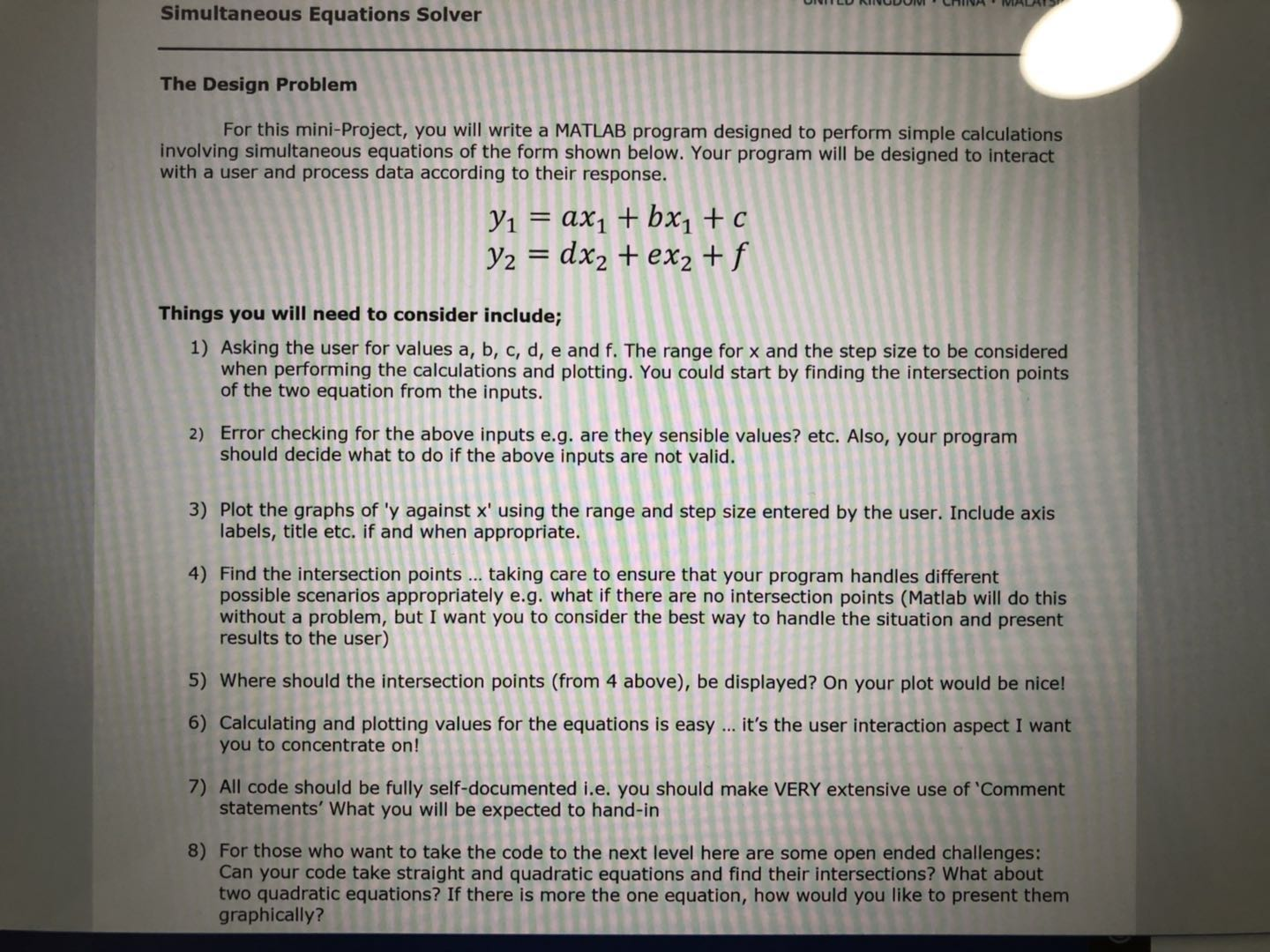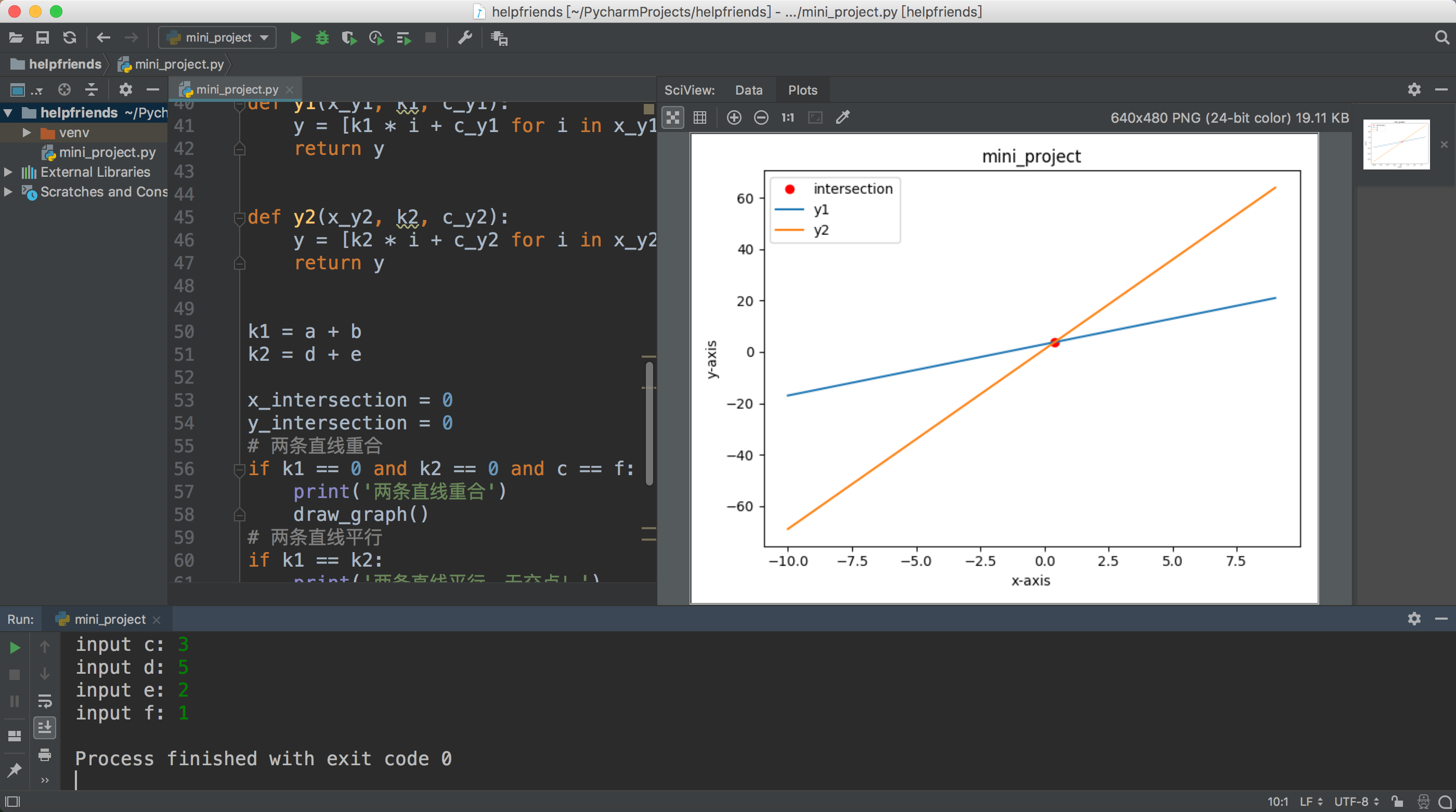• 统计来源于实际，在生活中有着广泛的应用。因此，统计的学习也应当从学生的实际 出发， 通过具体的案例， 通过对... 经过以上两步，我们就可以由采样点数据得到一条拟合直线，并得到其线性回归方程。 大家不妨试一试！
统计来源于实际，在生活中有着广泛的应用。因此，统计的学习也应当从学生的实际
出发，
通过具体的案例，
通过对现实问题的思考，
来充分调动他们积极性，
让学生意识到统
计就在我们身边，本功能需要使用
Excel
扩展功能，如果您的
Excel
尚未安装数据分析，请
依次选择“工具”
-
“加载宏”，然后选择加载“分析工具库”（
2003
版）。加载成功后，
可以在“工具”下拉菜单中看到“数据分析”选项。
（
1
）利用数据生成“
X
，
Y
散列点图”
选中数据，再依次选择“插入”
-
“图表”，选择
X,Y
散列点图
（
2
）添加趋势线
单击新生成的
X,Y
散列点图，
在依次选择工具栏按钮
“添加趋势线”
-
“类型”
中选
“线
性”，“选项”选择“显示公式”和“显示
R
平方值”两项。
如果工具栏没有“添加趋势线”按钮，可在菜单栏中选“视图”，然后依次选择“工
具栏”
-
“自定义”，在弹出的对话框中“命令”菜单下，在左侧“类别”选择中“制作图
表”，在右侧“命令”中将“添加趋势线”命令拖到工具栏。
经过以上两步，我们就可以由采样点数据得到一条拟合直线，并得到其线性回归方程。
大家不妨试一试！
展开全文• # 根据已知两点坐标，过这两点的直线解析方程： a*x+b*y+c = 0 (a >= 0) def getLinearEquation(p1x, p1y, p2x, p2y): sign = 1 a = p2y - p1y if a < 0: sign = -1 a = sign * a b = sign * (p1x - ...
# 根据已知两点坐标，求过这两点的直线解析方程： a*x+b*y+c = 0  (a >= 0)
def getLinearEquation(p1x, p1y, p2x, p2y):
sign = 1
a = p2y - p1y
if a < 0:
sign = -1
a = sign * a
b = sign * (p1x - p2x)
c = sign * (p1y * p2x - p1x * p2y)
return [a, b, c]

# 根据直线的起点与终点计算出平行距离D的平行线的方程
def getLinearEquation(p1x, p1y, p2x, p2y, distance):
"""
:param p1x: 起点X
:param p1y: 起点Y
:param p2x: 终点X
:param p2y: 终点Y
:param distance: 平距
:param left_right: 向左还是向右
"""
e = getLinearEquation(p1x, p1y, p2x, p2y)
f = distance * math.sqrt(e.a * e.a + e.b * e.b)
m1 = e.c + f
m2 = e.c - f
# result = 值1 if 条件 else 值2
c2 = m1 if p2y - p1y < 0 else m2
return [e.a, e.b, c2]
展开全文• 本人数学渣渣，公式来自...用方程法，避开了算K，真是太强了x2。 def calc_abc_from_line_2d(x0, y0, x1, y1): a = y0 - y1 b = x1 - x0 c = x0*y1 - x1*y0 return a, b, c def get_line_cross_point(line1, li
本人数学渣渣，公式来自知乎大佬，大佬真是太强了。 https://www.zhihu.com/question/381406535/answer/1095948349
用方程法，避开了算K，真是太强了x2。
def calc_abc_from_line_2d(x0, y0, x1, y1):
a = y0 - y1
b = x1 - x0
c = x0*y1 - x1*y0
return a, b, c

def get_line_cross_point(line1, line2):
# x1y1x2y2
a0, b0, c0 = calc_abc_from_line_2d(*line1)
a1, b1, c1 = calc_abc_from_line_2d(*line2)
D = a0 * b1 - a1 * b0
if D == 0:
return None
x = (b0 * c1 - b1 * c0) / D
y = (a1 * c0 - a0 * c1) / D
# print(x, y)
return x, y

if __name__ == '__main__':
# x1y1x2y2
line1 = [0, 5, 0, 10]
line2 = [5, 0, 10, 0]
cross_pt = get_line_cross_point(line1, line2)
print(cross_pt)


展开全文• 主要为大家详细介绍了python绘制直线的方法，绘制直线通用方法，具有一定的参考价值，感兴趣的小伙伴们可以参考一下
• def f(x,y): n=len(x) mx=sum(x)/n my=sum(y)/n sum_xy=sum([x[i]*y[i] for i in range(n)]) sum_xx=sum([x[i]*x[i] for i in range(n)]) b=(sum_xy-n*mx*my)/(sum_xx-n*mx*mx) a=my-b*mx ...python
• python3代码实现： &quot;&quot;&quot; y1 = a * x1 + b * x1 + c = (a+b) * x1 + c y2 = d * x2 + e * x2 + f = (d+e) * x2 + f ## 条件约束： 1. 用户输入a,b,c,d,e,f来得到两个方程的交点 ...

帮一位英国留学的小伙伴写的作业

题目要求：python3代码实现：
"""
y1 = a * x1 + b * x1 + c = (a+b) * x1 + c
y2 = d * x2 + e * x2 + f = (d+e) * x2 + f
## 条件约束：
1. 用户输入a,b,c,d,e,f来得到两个方程的交点
2. 对于用户的输入进行错误异常处理
3. 画坐标系以及两条直线方程
4. 找到交点，确保程序能够对不同的情形进行处理，例如没有交点，即两条直线平行的情况
5. 找到的交点，能够在图上突出的展示出来最好了
6. 关注用户交互方面
7. 归档，并及时交作业
8. 更多挑战，可以尝试考虑求二次方程的交点
"""

import numpy as np
import matplotlib.pyplot as plt

a = int(input('input a: '))
b = int(input('input b: '))
c = int(input('input c: '))
d = int(input('input d: '))
e = int(input('input e: '))
f = int(input('input f: '))

# 创建x坐标
x = np.arange(-10, 10, 1)

def draw_graph():
plt.title('mini_project')
plt.plot(x, y1(x, k1, c), label='y1')
plt.plot(x, y2(x, k2, f), label='y2')
# plt.plot(x_intersection, y_intersection, 'ro', label='intersection')
plt.xlabel('x-axis')
plt.ylabel('y-axis')
plt.legend()
plt.show()

def y1(x_y1, k1, c_y1):
y = [k1 * i + c_y1 for i in x_y1]
return y

def y2(x_y2, k2, c_y2):
y = [k2 * i + c_y2 for i in x_y2]
return y

k1 = a + b
k2 = d + e

x_intersection = 0
y_intersection = 0
# 两条直线重合
if k1 == 0 and k2 == 0 and c == f:
print('两条直线重合')
draw_graph()
# 两条直线平行
if k1 == k2:
print('两条直线平行，无交点！')
# 有交点：
if k1 != k2:
x_intersection = (f - c) / (k1 - k2)
y_intersection = k1 * x_intersection + c
plt.plot(x_intersection, y_intersection, 'ro', label='intersection')
draw_graph()


实现结果：展开全文• Python实现笛卡尔乘积的方法本文实例讲述了Python实现笛卡尔乘积的方法。分享给大家供大家参考，具体如下：在数学中，两个集合X和Y的笛卡尓乘积（Cartesian product），又称直积，表示为X × Y，第一个对象是X的...
• 霍夫变换直线检测目标前言1. 什么是霍夫变换直线检测2....学习使用OpenCV的Python接口调用函数：cv.HoughLines()，cv.HoughLinesP() 前言 霍夫变换是一种特征检测(feature extraction)，被广泛应用在图像分析（imaopencv 计算机视觉
• ## Python画直线

万次阅读 2014-06-25 19:09:01
import matplotlib.pyplot as plt import numpy as np beita = 1 gama = 0.5 x = np.linspace(0, 4, 1000) xx = x*9.8*0.6 OH = (0.33/(1-0.33)+beita)*(14.12-0.73*4.68)+0.73*4.68 Oh = (0.33/(1-0.33)+gama)*(14.
• 已知直线上两个端点坐标，任意等分点坐标，用什么方法或模块能实现?
• python方程的方法之一，sympy库。 巧妙转换也可解决问题。
• 知识点8一元二次方程根的判别式的应用【题目预览】【视频讲解】【分析点评】此题考查一元二次方程根的判别式与一元二次方程根的情况，当判别式的值大于0时，方程有两个不相等的实数根，当判别式的值等于0时，方程有...
• Python求两点之间的直线距离(2种实现方法)方法一：#导入math包import math#定义点的函数class Point:def __init__(self,x=0,y=0):self.x=xself.y=ydef getx(self):return self.xdef gety(self):return self.y#定义...
• 这篇文章主要介绍了python matplotlib拟合直线的实现,文中通过示例代码介绍的非常详细，对大家的学习或者工作具有一定的参考学习价值,需要的朋友可以参考下 代码如下 import numpy as np import matplotlib.pyplot ...
• ## python求线性回归

千次阅读 2017-01-05 10:23:47
线性回归python 线性回归的函数是 linregress(x,y) 函数； slope是斜率， intercept是截距， r_value 是相关系数from scipy import stats import numpy as np import pylabx = np.array([1, 2, 5, 7, 10, 15]) ...线性回归
• 常用的直线方程有一般式 点斜式 截距式 斜截式 两点式等等。除了一般式方程，它们要么不能支持所有情况下的直线（比如跟坐标轴垂直或者平行），要么不能支持所有情况下的点（比如x坐标相等，或者y坐标相等）。...图形 计算几何
• 本文要解决如下问题：给定一些三维空间点，要求用直线拟合这些点，直线方程直线方程的三种表示方法： 1.一般式： 它实际上表示，直线是由经验分享
• 两点间距离 /***** 两点间距离*****/ float getDistance(CvPoint pointO, CvPoint pointA) { float distance; distance = powf((pointO.x - pointA.x), 2) + powf((pointO.y - ...点到直线的距离:P到AB的距离
• 一条直线3.测试枚举线段点4.完整测试代码 实例图片镇楼 1.读取图片,阈值处理 # 读取图片并灰度值化 img = cv2.imread('d:1.jpg', 0) # 二值化,过滤掉噪音 img = np.where(img < 100, 0, 255).astype(np.uint8) ...opencv
• 设任意两个有序点P、Q对应于n维矢量空间中的一个矢量a，那么过两点的直线方程为：（1-t)P + tQ笛卡尔平面是一个仿射空间，那么空间两点（a,b), (c, d)之间的参数化方程为：L = ｛((1-t)a + tc, (1-t)b + td) | t是...图形 3d
• 应用题目:已知梯形的高是7，面积是56 cm2，它的上底比下底的三分之一多4 cm，该梯形的上底和下底的长度分别是多少？ 3.解题思路:假设上底为长度为x_0 cm,下底为x_1 cm,高为h cm,面积为s cm2,那么由题意可知: h=7...
• 最近在做图像的透射变换，有一个关键问题就是找到原图像内四边形的四个顶点...首先，我们定义：直线L1：[x1,y1,x2,y2]，直线L2：[x3,y3,x4,y4]简单解释一下原理：两点确定一条直线，而每一条直线都可以表示为：Y=kX+...
• 而这时候，我们会采取将手指两边中点拟合成一条直线，求得这个直线的直线方程，然后得到旋转的角度，以便对原来的图像进行旋转操作。当我们知道如何取得手指的边缘中点后，直接用fitLine函数可以方便的按我们想要的...cv.fitLine
• 文章目录 需求：在图像上将检测到的线段转换为过图像边界的直线 def myline(x1,x2,y1,y2,w,h): """ 线段的端点转换为图像的边界点 :param x1: 已知线段的2个端点 :param x2: :param y1: :param y2: :param w: 图像的...
• Code（python） x = [] y = [] n = input(); n = int(n) sumx = 0 sumx2 = 0 sumy = 0 sumxy = 0 for i in range(n): v = input() v = float(v) sumx += v sumx2 += (v * v) x.append(v)...
• 中国大学慕课2020Python编程基础答案搜题公众号更多相关问题如图所示，一定量的空气，开始在状态A，其压强为2.0×105Pa，体积为2.0×10－3m3，沿直线AB变化到状态B后，压强变为1如果一个等腰三角形的周长为28厘米，...
• Python最小二乘法拟合直线，采用了2种不同的方式，一种是直接计算，另一种是调用numpy.linalg.solve()
• 一、 先说我对这个题目的理解直线的x，y方程是这样的：y = kx+b， k就是斜率.线性回归斜率， 就是说 有这么一组(x, y)的对应值——样本。如果有四组，就说样本量是4.根据这些样本，做“线性回归”，最终出一条......

# python求直线方程python 订阅